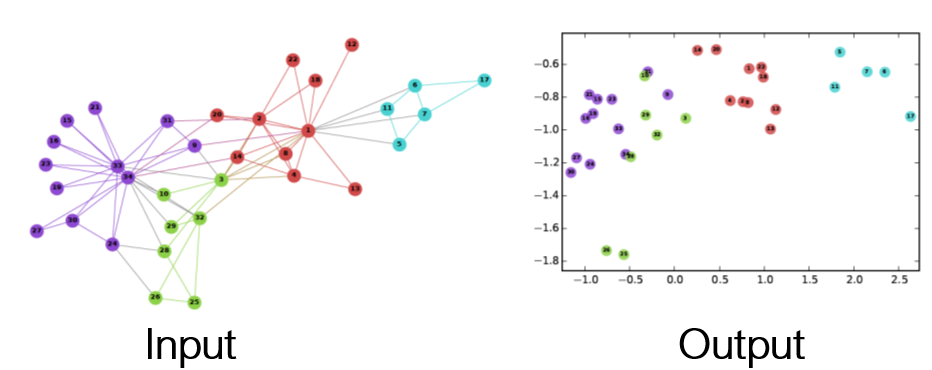# Node2Vec — Graph Embedding Method

Source: Deep Learning on Medium# Node2Vec — Graph Embedding Method

Graphs are common data structures to represent information with connections. For example, Protein-Protein Interaction, where nodes represent proteins, and an edge indicates a biological interaction between a pair of proteins. Another example, is Facebook network where nodes are users and edges are connections between the users.

If we want to make predictions on those graphs using deep learning methods, we need a way to transform them into a d-dimensional vector of real number. So, we use graph embeddings, a low dimension representations which help generalize better the input data.

In Node2Vec  we aim to preserve network neighborhoods of nodes, so our goal is to find a representation of the graph as a real vector, where neighborhoods have small euclidean distance between them and at the same time the node structure is represented.

In order to understand the concept of Node2Vec we first need to learn how Word2Vec works. Notice that sentences are a special case of a directed graph with one-degree nodes. There are several ways to produce word embeddings, which are a condense representation of words. In Word2Vec SGNS- skip-gram (context representation) negative sampling (training method) we predict the context based on the word. We want the probability of having the correct word in the context to be high and the probability of having a random word in the context to be low. For example:

(The, baby, is, smiling, at, her, mom) — we will give high score to predict context “the baby is ___ at her mom”
(The, baby, is, flying, at, her, mom) — we will give low score to predict context “the baby is ___ at her mom”

How do we build the tuples to give as input to our algorithm?
This is a very important question. The way we build the samples form which we learn the embedding, effects the embedding space. For example using content words which are connected to the target word by a dependency edge will emphasis on similarity between word functionality. Where as using content words of a small window around the target words (CBOW) will emphasis on topical similarities .

Now lets get back to Node2Vec, we want to find a way to create those tuples. Our target is to preserve neighborhoods and structures, therefore we need a compatible way to explore the graph. We use a second order random walk in order to sample the graph that trades-off between BFS and DPS, between exploring the neighbors and exploring other neighborhoods, between micro-view and macro-view.

The trade-off is achieved by using hyper-parameters p and q to calculate the unnormalized transition probability.
p — return rate
q — exploration rate
Assuming we walked from t to v and now we need to decide which node x_i to explore. We calculate the number of edges between t and the possible x_i nodes. If the distance is zero (meaning it’s t itself) we multiply the original edge weight by 1/p. If the distance is 1 we keep the original edge weight. If the distance is 2 we multiply the edge weight by 1/q. Notice that low p / q leads to high return probability / exploration probability.

To sum up, Node2Vec defines a smart way to explore the graph, such that homophily and structural equivalence are embodied in the representation, in order to create samples for the SGNS algorithm.

References:

 Grover & Leskovec, node2vec: Scalable Feature Learning for Networks
 Levy & Goldberg, Dependency-Based Word Embeddings